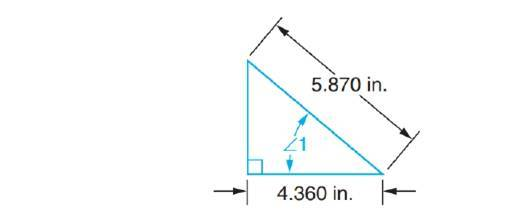# Solve the following exercises. Compute angles to the nearer minute in triangles with customary unit sides. Compute angles to the nearer hundredth degree in triangles with metric unit sides. Determine ∠ 1.FindFindarrow_forward

### Mathematics For Machine Technology

8th Edition
Peterson + 1 other
Publisher: Cengage Learning,
ISBN: 9781337798310

#### Solutions

Chapter
SectionFindFindarrow_forward

### Mathematics For Machine Technology

8th Edition
Peterson + 1 other
Publisher: Cengage Learning,
ISBN: 9781337798310
Chapter 68, Problem 9A
Textbook Problem
1 views

## Solve the following exercises. Compute angles to the nearer minute in triangles with customary unit sides. Compute angles to the nearer hundredth degree in triangles with metric unit sides. Determine ∠ 1.To determine

The value of angle 1.

### Explanation of Solution

Hypotenuse = 5.870 in

From the given triangle,

Now,

1°=1360ofacircle1=160

### Still sussing out bartleby?

Check out a sample textbook solution.

See a sample solution

#### The Solution to Your Study Problems

Bartleby provides explanations to thousands of textbook problems written by our experts, many with advanced degrees!

Get Started

Find more solutions based on key concepts
In Exercise 9-16, find the steady state vector for the transition matrix. 

Finite Mathematics for the Managerial, Life, and Social Sciences

In Exercises 90-98, determine whether the statement is true or false. If it is true, explain why it is true. If...

Applied Calculus for the Managerial, Life, and Social Sciences: A Brief Approach

In Exercises 5-10, solve for y in terms of x. 4x+y=7

Calculus: An Applied Approach (MindTap Course List)

Write the number in the form a + bi. 41. ei/2

Single Variable Calculus: Early Transcendentals, Volume I

It does not exist.

Study Guide for Stewart's Multivariable Calculus, 8th

Graphing Inequalities 16. y 1 x

Precalculus: Mathematics for Calculus (Standalone Book)

Use the Taylor polynomial of degree 2 for centered at 1 to estimate . 1.250 1.255 1.265 1.270

Study Guide for Stewart's Single Variable Calculus: Early Transcendentals, 8th

Find the slope of the line through P and Q. 10. P(1, 4), Q(6, 0)

Single Variable Calculus: Early Transcendentals